Thermodynamics and Propulsion

All bodies radiate energy in the form of photons moving in a random direction, with random phase and frequency. When radiated photons reach another surface, they may either be absorbed, reflected or transmitted. The behavior of a surface with radiation incident upon it can be described by the following quantities:

•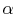= absorptance - fraction of incident radiation absorbed
•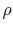= reflectance - fraction of incident radiation reflected
•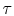= transmittance - fraction of incident radiation transmitted.
Figure 19.1 shows these processes graphically.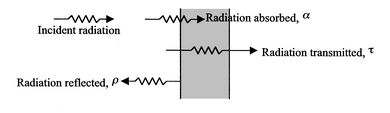From energy considerations the three coefficients must sum to unity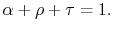Reflective energy may be either diffuse or specular (mirror-like). Diffuse reflections are independent of the incident radiation angle. For specular reflections, the reflection angle equals the angle of incidence.

Subsections
UnifiedTP